Varsity Tutors always has a different ACT Math Question of the Day ready at your disposal! If you’re just looking to get a quick review into your busy day, our ACT Math Question of the Day is the perfect option. Answer enough of our ACT Math Question of the Day problems and you’ll be ready to ace the next test. Check out what today’s ACT Math Question of the Day is below.

### Question of the Day: ACT Math

What is the surface area of a composite figure of a cone and a sphere, both with a radius of 5 cm, if the height of the cone is 12 cm? Consider an ice cream cone as an example of the composite figure, where half of the sphere is above the edge of the cone.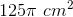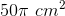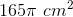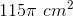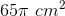The ACT Mathematics section is one of five components of the ACT exam, and tests high school students’ aptitude in mathematical reasoning. The test features practical questions in Pre-Algebra, Elementary Algebra, Intermediate Algebra, Coordinate and Plane Geometry, and Trigonometry. Whether you need ACT Math tutoring in New YorkACT Math tutoring in Chicago, or ACT Math tutoring in Los Angeles, working one-on-one with an expert may be just the boost your studies need.

When taking the ACT Math section, students have one hour to answer sixty questions. This works out to one minute per question, but some questions will take more time, particularly word problems and questions requiring calculation, while others should take less than a minute, or even just a few seconds. Most students who perform well on the Math portion of the ACT have taken ACT math practice tests; they have also likely become familiar with how questions are framed, as well as tricks and traps ACT question-writers use to test comprehension and mathematical reasoning. In addition, high scorers may take the time to practice the various ways of reaching answers: calculation, educated guessing, and translating questions into plain English. To help you prepare you may want to consider ACT Math tutoring, as well our free ACT Math flashcards.

The good news is that the ACT does not test advanced high school mathematics like calculus; the bulk of the Math section’s questions are related to various levels of algebra and geometry, while some problems feature trigonometry. Questions can cover any component of these math subjects, from probability and exponents to quadratic equations and slopes of lines.

While the other multiple-choice sections of the ACT feature four answer options, the Math section’s questions each have five options. So, in light of probability, there is less chance of one arriving at the correct answer by guessing if one has not narrowed down the number of answer choices beforehand. Often the answers will be numerical, but some questions will involve choosing the correct equation or expression. Questions may be made more difficult by using complex language, leaving out geometrical figures, or hiding the mathematical operation within a word problem. Such formatting and phrasing choices can make even pre-algebra questions challenging.

Many students find it helpful to answer questions out of order, first scanning the questions to see if there are any easy ones, completing those, and moving on to the more difficult and time-consuming questions. A question can be easy if it requires little calculation, seems straightforward, or tests a concept about which a student feels particularly confident, while difficult questions look as if they require minutes of time to interpret and answer, are confusing, or are outside of a student’s comfort zone.

Should you finish the ACT Math section before time is called, it is important to spend the remaining minutes checking your work and choosing answers for difficult questions based on the work you have done on those problems. There is no credit given for unmarked questions and the ACT does not deduct points for wrong answers, so guessing can only boost your score should you get a few questions right in this manner. You may want to try out practice exams which mimic the conditions of the test, completing each section within the same time allotted as the real exam. This may help you adjust your pacing in each section and move quickly from the end of one test to the start of another. After all, there’s no reason to be hung up on a difficult math question when the Math section is over and you are working on a completely different section. In addition to an ACT Math tutor you may benefit from using our free ACT prep book as well.

If you want to start working on your ACT Math knowledge right now, our Question of the Day can help you do so. Every day, Varsity Tutors features a different multiple-choice ACT Math question drawn from one of the many topics which can appear on the exam. Once you choose your response, the answer to the question is revealed, as well as a comprehensive explanation of how to arrive at it. If you miss the question, consider it a valuable learning opportunity: figuring out where you made a misstep in your approach means you are much less likely to make the same mistake again on test day. You can check back every day to test your skills with a new ACT math question, and explore our other free ACT Math resources to help you continue practicing!

### All ACT Math Resources